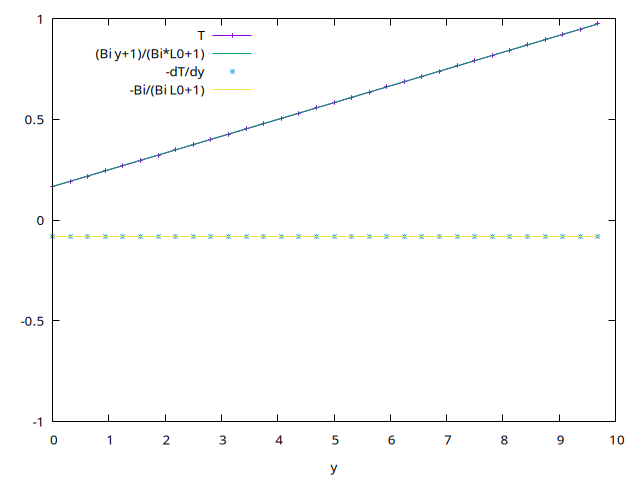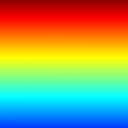# The Steady Heat Equation in a square

The Steady Heat Equation in a square for T, written without dimension \displaystyle \nabla^2 T = 0 with mixed condition on the wall y=0 for any x \displaystyle - \frac{\partial T }{\partial n} = Bi\; T where n is in the direction of the external normal, Bi is the Biot number, it is the ratio of the heat transfer coefficient h by conductivity k multiplied by the reference length L. So, if the boundary is at the bottom, the normal direction is reversed compared to the y direction \displaystyle \frac{\partial T }{\partial y} (x,0)= Bi\; T (x,0)

and at the top a fixed temperature T(x,top)=1, right and left are periodic conditions (or infinite domain),

Note that if Bi>>1 this gives the fixed wall temperature T=0.

This system can be solved with the Poisson solver.

#include "grid/multigrid.h"
#include "run.h"
#include "poisson.h"

Temperature and flux, and some obvious variables

scalar T[],flux[];
double Bi,tmax,dy;

The generic time loop needs a timestep. We will store the statistics on the diffusion solvers in mgdpoi.

mgstats mgpoi;

double Texact(double y)
{ double Te;
Te = (Bi*y+1)/(Bi*L0+1);
return Te;
}

Boundary conditions: a given temperature at the top, a mixed convection at the bottom \partial T/\partial y = Bi\; T this mixed condition is written (T[0,0]-T[bottom])/Delta = Bi (T[0,0] + T[bottom])/2

T[top]    =  dirichlet(1);
T[bottom] =  T[]*(2.-Bi*Delta)/(2.+Bi*Delta) ;
T[right]  =  neumann(0);
T[left]   =  neumann(0);

## Parameters

The size of the domain L0.

int main() {
L0 = 10.;
X0 = -L0/2;
Y0 = 0;
N =  32;
tmax = 500;
Bi = .5;
run();
}

## Initial conditions

a constant amount of concentration

event init (i = 0) {
foreach()
T[] = 0.;
boundary ({T});
}

## integration

event integration (i++) {

prepare Poisson solver with zero source

  scalar source[];
foreach() {
source[] = 0;
}
mgpoi = poisson (T,source);

The flux along y is -{\partial c}/{\partial y}

  foreach()
flux[] =  - ( T[0,0] - T[0,-1] )/Delta;
boundary ({flux});

}

## Outputs

print data, saves along the center

event printdata ( i++) {
FILE *  fpx = fopen("T.txt", "w");

for (double y = 0 ; y < L0; y += L0/N){
fprintf (fpx, "%g %g %g \n",
y, interpolate (T, 0, y) , interpolate (flux, 0, y));}

fclose(fpx);

}

At the end of the simulation, we create snapshot images of the field, in PNG format.

event pictures (i++) {
output_ppm (T, file = "T.png", min=0, max = 1,  spread = 2, n= 128, linear = true);
}

## Run

Then compile and run:

rm heat2D; qcc  -g -O2 -DTRASH=1 -Wall heat2D.c -o heat2D ; ./heat2D 

or with make

 ln -s ../../Makefile Makefile
make heat2D.tst;make heat2D/plots
make heat2D.c.html ; open heat2D.c.html 

## Results

compare a cut in x=0, exact solution and computed

set output 'plot.png'
Bi=.5
L0=10
set xlabel "y"
set key left
p[][-1:1]'T.txt't 'T'w lp,(Bi*x+1)/(Bi*L0+1) t'(Bi y+1)/(Bi*L0+1)','T.txt'u 1:3 t'-dT/dy',1 not,-Bi/(Bi*L0+1.) t '-Bi/(Bi L0+1)'cut in x=0 (script)

Field of T:## Bibliography

More on the heat equation by PYL

Version 1: may 2014